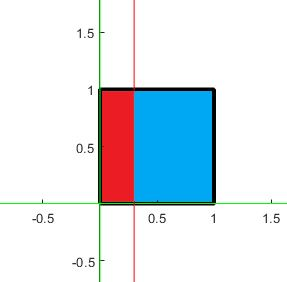# Problem 49903. Splitting Square - Problem the first

Consider a square sitting in Quadrant I as depicted in an example below:This square is to be split into two regions (e.g., red and blue). Given the ratio between the two regions and the side of the square, determine the x coordinate of the line that splits the regions. The ratio between the regions (red to blue) is presented through the first two entries in the input. For example, if the ratio is 7 to 11, then these two numbers will be the first two numbers in the input. The last entry is the side of the square.

### Solution Stats

31.4% Correct | 68.6% Incorrect
Last Solution submitted on Aug 23, 2023

### Community Treasure Hunt

Find the treasures in MATLAB Central and discover how the community can help you!

Start Hunting!